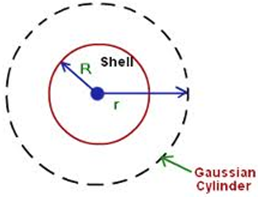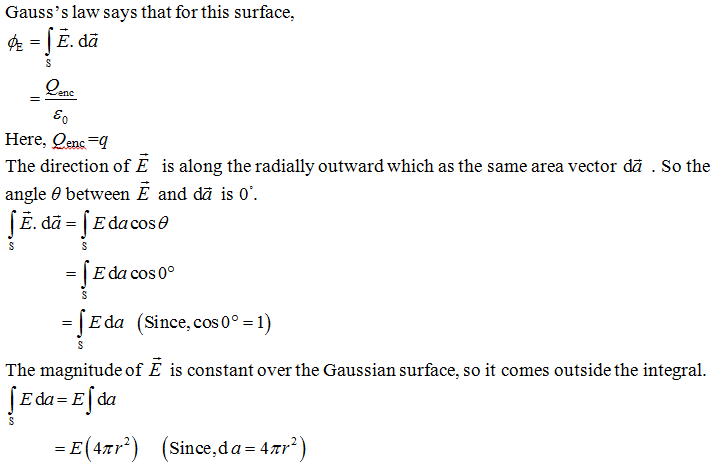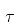Courses

# Notes | EduRev

## JEE Main Mock Test Series 2020 & Previous Year Papers

Created by: Learners Habitat

## JEE : Notes | EduRev

The document Notes | EduRev is a part of the JEE Course JEE Main Mock Test Series 2020 & Previous Year Papers.
All you need of JEE at this link: JEE

Question 1: An electrically neutral penny, of mass m = 3.11 g, contains equal amounts of positive and negative charge.
(a) Assuming that the penny is made entirely of copper, what is the magnitude q of the total positive (or negative) charge in the coin?
(b) Suppose that the positive charge and the negative charge in a penny could be concentrated into two separate bundles, 100 m apart. What attractive forces would act on each bundle?
Solution:-
(a) A neutral atom has a negative charge of magnitude Ze associated with its electrons and a positive charge of the same magnitude associated with the protons in its nucleus, where Z is the atomic number of the element in question. For copper, Z= 29, which means that an atom of copper has 29 protons and, when neutral, 29 electrons.
The charge magnitude q we seek is equal to NZe, in which N is the number of atoms in the penny. To find N, we multiply the number of moles of copper in penny by the number of atoms in a mole (Avogadro’s number, NA=6.02×1023 atoms/mol). The number of moles of copper is 63.5 g/mol. Thus we have
N= N(m/M ) = (6.02×1023 atoms/mol) (3.11 g/63.5 g/mol)
= 2.95×1022 atoms
We then find the magnitude of the total positive or negative charge in the penny to be,
q = NZe
= (2.95×1022) (29) (1.60×10-19C)
=137,000 C
This is an enormous charge. (For comparison, if you rub a plastic rod with fur, you will be lucky to deposit any more than 10-9 C on the rod.)
(b) We have,
F = (1/4πε0) (q2/r2)
= (8.99×109 N.m2/C2) (1.37×105 C)2/(100 m)2
= 1.69×1016 N
From the above observation we conclude that, the attractive force act on each bundle would be 1.69×1016 N.

Question 2: Find the field outside a charged solid sphere of radius R and total charge q.
Solution:
Draw a spherical surface of radius r>R in the below figure. This is called Gaussian surface.From the above observation we conclude that, the field outside the sphere is exactly the same as it would have been if all the charge had been concentrated at the center.

Question 3: A neutral water molecule (H2O) in its vapor state has an electric dipole moment of 6.2×10-30C.m.
(a) How far apart are the molecule’s centers of positive and negative charge?
(b) If the molecule is placed in an electric field 1.5×104 N/C, what maximum torque can the field exert on it?
(c) How much work must an external agent do to turn this molecule end for end in this field, starting from its fully aligned position, for which θ = 0?
Solution:
(a) There are 10 electrons and 10 protons in this molecule. So the magnitude of the dipole moment is
p = qd = (10e)(d)
In which d is the separation we are seeking and e is the elementary charge. Thus
d = p/10e
= [6.2×10-30 C.m/(10) (1.60×10-19C)]
= 3.9×10-12 m = 3.9 pm
This distance is not only small, but it is actually smaller than the radius of a hydrogen atom.
(b) From the equation= pE sinθ we know that the torque is a maximum when θ = 90°. Substituting this value in that equation gives,= pE sinθ
= (6.2×10-30 C.m) (1.5×10N/C) (sin 90°)
= 9.3×10-26 N.m
Therefore, the maximum torque would be 9.3×10-26 N.m
(c) The work is the difference in potential energy between the positions θ = 180° and θ =0. Using equation U = -pE cosθ, we get,
W= U (180°) – U(0)
= (-pE cos180°) – (- pE cos 0)
= 2pE
= 2(6.2×10-30 C.m) (1.5×10N/C)
=1.9×10-25 J
From the above observation we conclude that, the work done by the external agent would be 1.9×10-25 J.

Question 4: The electrostatic repulsive force between two positively charged ions carrying equal charges is given by 3.7×10 N. These charges are separated by a distance of 5×10-10 m. Calculate the number of electrons missing from each ion?
Solution:
It is given that, F=3.7×10-9 N, r =5×10-10 m and q1=q=q
According to Coulomb's law,
F=(9×109) (q1q2/r2)
3.7 × 10-9=9 ×109× [q2/(5×10-10)2]
q2=[3.7×10-9×(5×10-10)2] / (9×109)
q2=10.28×10-38 C
q=3.2×10-19C
The charge of the electron is given by 1.6×10-19C
Therefore, number of electrons missing from each ion
=Total charge of each electron
= (3.2×10-19)/ (1.6×10-19)
=2
From the above observation we conclude that, the number of electrons missing from each ion will be 2.

Offer running on EduRev: Apply code STAYHOME200 to get INR 200 off on our premium plan EduRev Infinity!

## JEE Main Mock Test Series 2020 & Previous Year Papers

3 videos|174 docs|149 tests

,

,

,

,

,

,

,

,

,

,

,

,

,

,

,

,

,

,

,

,

,

;